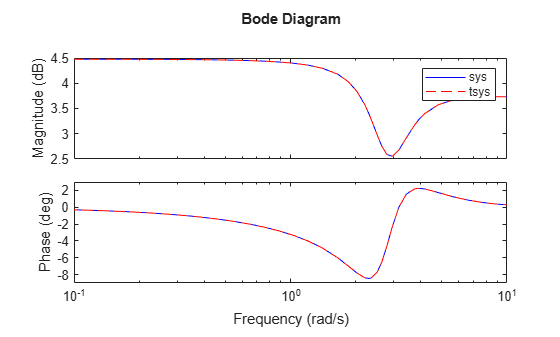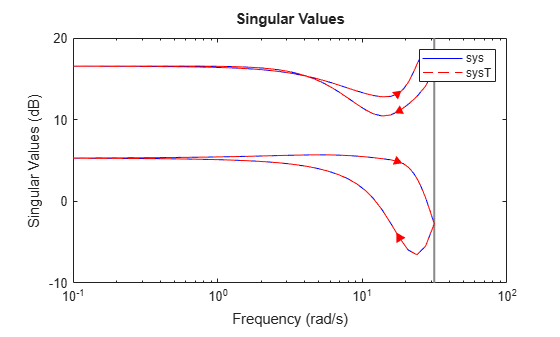ss2ss

State coordinate transformation for state-space model

Description

ss2ss performs the similarity transformation z = Tx on the state vector x of a state-space model. For more information, see Algorithms.

example

sysT = ss2ss(sys,T) performs the state-coordinate transformation of sys using the specified transformation matrix T. The matrix T must be invertible.

Examples

collapse all

Perform a similarity transform for a state space model.

Generate a random state-space model and a transformation matrix.

rng(0)
t = randn(5);

Perform the transformation and plot the frequency response of both models.

tsys = ss2ss(sys,t);
bode(sys,'b',tsys,'r--')
legendThe responses of both models match closely.

ss2ss applies state transformation only to the state vectors of the numeric portion of the generalized model.

Create a genss model.

sys = rss(2,2,2) * tunableSS('a',2,2,3) + tunableGain('b',2,3)
sys =

Generalized continuous-time state-space model with 2 outputs, 3 inputs, 4 states, and the following blocks:
a: Tunable 2x3 state-space model, 2 states, 1 occurrences.
b: Tunable 2x3 gain, 1 occurrences.

Type "ss(sys)" to see the current value, "get(sys)" to see all properties, and "sys.Blocks" to interact with the blocks.

Specify a transformation matrix and obtain the transformation.

T = [1 -2;3 5];
tsys = ss2ss(sys,T)
tsys =

Generalized continuous-time state-space model with 2 outputs, 3 inputs, 4 states, and the following blocks:
a: Tunable 2x3 state-space model, 2 states, 1 occurrences.
b: Tunable 2x3 gain, 1 occurrences.

Type "ss(tsys)" to see the current value, "get(tsys)" to see all properties, and "tsys.Blocks" to interact with the blocks.

Decompose both models.

[H,B,~,~] = getLFTModel(sys);
[H1,B1,~,~] = getLFTModel(tsys);

Obtain the transformation separately on the model from decomposed sys.

H2 = ss2ss(H,T);

Compare this transformed model with the model from decomposed tsys.

isequal(H1,H2)
ans = logical
1

Both models are equal.

The file icEngine.mat contains one data set with 1500 input-output samples collected at the a sampling rate of 0.04 seconds. The input u(t) is the voltage (V) controlling the By-Pass Idle Air Valve (BPAV), and the output y(t) is the engine speed (RPM/100).

Use the data in icEngine.mat to create a state-space model with identifiable parameters.

z = iddata(y,u,0.04);
sys = n4sid(z,4,'InputDelay',2);

Specify a random transformation matrix.

T = randn(4);

Obtain the transformation.

sysT = ss2ss(sys,T);

Compare the frequency responses.

bode(sys,'b',sysT,'r--')
legendThe responses match closely.

ss2ss also lets you perform similarity transformation for models with complex coefficients.

For this example, generate a random state-space model with complex coefficients.

rng(0)
sys = ss(randn(5)+1i*randn(5),randn(5,3),randn(2,5)+1i*randn(2,5),0,.1);

Specify a transformation matrix containing complex data.

T = randn(5)+1i*randn(5);

Obtain the transformation.

sysT = ss2ss(sys,T);

Compare the singular values of the frequency response.

sigma(sys,'b',sysT,'r--')
legendThe responses match closely for both branches.

Input Arguments

collapse all

Dynamic system, specified as a SISO, or MIMO dynamic system model. Dynamic systems that you can use include:

• Continuous-time or discrete-time numeric LTI models, such as ss or dss models.

• Generalized or uncertain LTI models, such as genss or uss (Robust Control Toolbox) models. (Using uncertain models requires Robust Control Toolbox™ software.)

For such models, the state transformation is applied only to the state vectors of the numeric portion of the model. For more information about decomposition of these models, see getLFTModel and Internal Structure of Generalized Models.

• Identified state-space idss (System Identification Toolbox) models. (Using identified models requires System Identification Toolbox™ software.)

If sys is an array of state-space models, ss2ss applies the transformation T to each individual model in the array.

Transformation matrix, specified as an n-by-n matrix, where n is the number of states. T is the transformation between the state vector of the state-space model sys and the state vector of the transformed model sysT. (See Algorithms.)

Output Arguments

collapse all

Transformed state-space model, returned as a dynamic system model of the same type as sys.

Algorithms

ss2ss performs the similarity transformation $\overline{x}=Tx$ on the state vector x of a state-space model.

This table summarizes the transformations returned by ss2ss for each model form.

Input ModelTransformed Model

Explicit state-space models of the form:

$\begin{array}{l}\stackrel{˙}{x}=Ax+Bu\\ y=Cx+Du\end{array}$

$\begin{array}{l}\stackrel{˙}{\overline{x}}=TA{T}^{-1}\overline{x}+TBu\\ y=C{T}^{-1}\overline{x}+Du\end{array}$

Descriptor (implicit) state-space models for the form:

$\begin{array}{c}E\stackrel{˙}{x}=Ax+Bu\\ y=Cx+Du\end{array}$

$\begin{array}{c}E{T}^{-1}\stackrel{˙}{\overline{x}}=A{T}^{-1}\overline{x}+Bu\\ y=C{T}^{-1}\overline{x}+Du\end{array}$

Identified state-space (idss) models of the form:

$\begin{array}{c}\frac{dx}{dt}=Ax+Bu+Ke\\ y=Cx+Du+e\end{array}$

$\begin{array}{l}\stackrel{˙}{\overline{x}}=TA{T}^{-}{}^{1}\overline{x}+TBu+TKe\\ y=C{T}^{-}{}^{1}\overline{x}+Du+e\end{array}$

Compatibility Considerations

expand all

Behavior changed in R2021b

Errors starting in R2021b